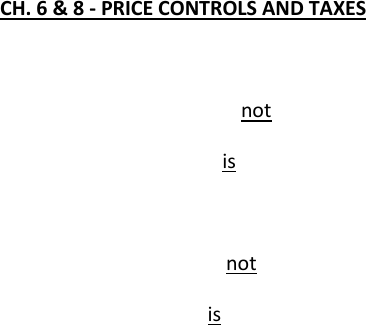Textbook Notes (270,000)
CA (160,000)
McMaster (10,000)
ECON (800)
ECON 1B03 (300)
Chapter 6-14

# ECON 1B03 Chapter Notes - Chapter 6-14: Perfect Competition, Takers, Market Power

Department
Economics
Course Code
ECON 1B03
Professor
Hannah Holmes
Chapter
6-14

Page:
of 9CH. 6 & 8 - PRICE CONTROLS AND TAXES
A price ceiling is a legal maximum on the price at which a good can be sold.
The price ceiling is not binding (not effective) if it is set above equilibrium price.
The price ceiling is binding (effective) if set below equilibrium price, leading to a shortage.
A price floor is a legal minimum on the price at which a good can be sold
The price floor is not binding if set below the equilibrium price.
The price floor is binding if set above the equilibrium price, leading to a surplus.
Inefficient allocation of sales
those willing to sell the good at a lower price aren’t always the ones that manage to sell it.
eg. You’re willing to work below min wage but don’t get hired while a student has a job she
doesn’t really care about but thinks the wage is worth it.
Wasted resources
You may spend a lot of time looking for work.
A quota is a quantity control.
An upper limit on the quantity of a good that can be sold.
The difference between these 2 prices is the quota rent: quota owners receive an additional
\$1.20 per litre per week. (9mill litres of milk per week - customer would pay 1.80 “demanded
price”, producers are happy with \$0.60 per litre)
This is also the VALUE of the quota
Tax incidence is the distribution of a tax burden.
In our example, the consumers bore the larger burden of the tax.
That’s because the demand curve is actually steeper than the supply curve.
The side of the market which is more inelastic (steeper curve) bears a larger burden of
the tax.
As the size of the tax rises, tax revenue grows.
But as the size of the tax continues to rise, tax revenue falls because the higher tax reduces the
size of the market.
CH. 13 - COSTS OF PRODUCTION
Profit
Profit = Total revenue Total cost
P = TR - TC
Total Revenue, TR
o The amount a firm receives for the sale of its output.
Total Cost, TC
o The market value of the inputs a firm uses in production.
Explicit and Implicit Costs
A firm’s cost of production include explicit costs and implicit costs.
Explicit costs are input costs that require a direct outlay of money by the firm (you have a bill for
your accountant).
Implicit costs are input costs that do not require an outlay of money by the firm (like the money
you could have earned investing instead of updating your assembly line) that is, opportunity
costs.
economic profit as total revenue minus total cost, including both explicit and implicit costs, i.e.,
opportunity costs.2122
accounting profit as the firm’s total revenue minus only the firm’s explicit costs.
When total revenue exceeds both explicit and implicit costs, the firm earns economic profit.
Economic profit is smaller than accounting profit because it includes implicit costs.
The production function shows the relationship between quantity of inputs used to make a good and
the quantity of output of that good
The marginal product of any input in the production process is the increase in output that arises
from an additional unit of that input.
MP = change in total output = Q
change in # of inputs L
*We use L (labour) here because it’s our only input.
The production function shows the relationship between quantity of inputs used to make a good
and the quantity of output of that good.
Diminishing marginal product is the property whereby the marginal product of an input declines
as the quantity of the input increases
often refer to diminishing MP as diminishing returns to an input.
TP is maximized when MP = 0 (slope = 0)
Fixed costs are those costs that do not vary with the quantity of output produced.
Examples: rent, loan payments, salaried administrative staff
Variable costs are those costs that do vary with the quantity of output produced.
Examples: labour costs, raw material costs
Short Run, SR: the period of time in which at least one input into production is fixed.
Long Run, LR: the period of time in which all inputs into production can vary.
Total Cost = Total Fixed Cost + Total Variable Cost
TC = TFC + TVC
If there are fixed costs, the time period must be the SR.
Marginal Cost, MC as the increase in total cost that arises from an extra unit of production.
MP = change in total cost = TC
change in total outputs Q
MC intersects AVC at min AVC.
MC intersects ATC at min ATC.
Whenever MC < AVC or ATC, AVC and ATC must be falling.
Whenever MC > AVC or ATC, AVC and ATC must be rising.
Average total cost declines as output increases.
Average total cost starts rising because average variable cost rises substantially
Min ATC is the point of efficient scale.
The MC curve is the “inverse” of the MP curve and ATC is the “inverse” of AP
For example, say Jerry hires an office manager whose salary increases his fixed costs.